# Background Researches¶

### (1) Gaming Character

The dataset of the gaming characters are attained by using web scrawler in python. The images are from: Mobafire

The shape of the chracters vary vastly, so the GAN model finds a bit hard to generate images that look like the real images perfectly.

### (2) Anime Character

The dataset of the the images are from: Kaggle

The shape of the chracters does not vary vastly, so the GAN model finds it easy to generate images that look like the real images perfectly.

### (3) Sketching Face

The dataset of the sketching faces are attained from kaggle. The images are from: Kaggle

The shape of the chracters does not vary vastly, so the GAN model finds a it easy to generate images that look like the real images perfectly. And the generated images looks more like a human face than the real dataset. However, during the first few epoches, the GAN model only generates blank images, unlike other datasets. This can be because as the image contains only black and white dots, it is easy for the model to learn the data distribution from all images and generate an image that looks more like human faces by combining all the features learnt. However, the discriminator is also very easy to distinguish real and fake images at the start, so that results in the generator learns very rare at the start, despite the discrimitor is not fully trained yet.

### (4) Real Faces

The dataset of the real faces are attained from kaggle. The images are from: Kaggle

The shape of the chracters does vary vastly, so the GAN model finds it very easy to generate images that look like the real images perfectly. One commonality for the dataset of faces is that they generate pictures that look all the same at the start, however they managed to learn more features at a later stage so the images generated at 200 epoches will be all different. However, for the gaming characters dataset, it generates images that look differently at the very early trainning stage of 10th epoches. This can be because the trainning datasets' difference.

GAN Performance Progress Visualization for Gaming Characters Training.

In [ ]:
from IPython.display import Image

Out[ ]:GAN Performance Progress Visualization for Anime Characters Training.

In [ ]:
Image(url='https://sgdatadog.com/anime.gif')

Out[ ]:GAN Performance Progress Visualization for Sketching Characters Training.

In [ ]:
Image(url='https://sgdatadog.com/sketch.gif')

Out[ ]:GAN Performance Progress Visualization for Real Pretty Face Training.

In [ ]:
Image(url='https://sgdatadog.com/real-retty.gif')

Out[ ]:# Content Overview¶

I. Importing Libraries.

II. Load Image Data From Google Dirve

III. Image Data Generator

IV. Define Discriminator

V. Define Generator

VI. Define GAN

VII. Trainning GAN

VIII. Visualize GAN Model Performance

IX. Sub-Section with more original data

• Anime Figures
• Pretty-Face (Sketch)
• Pretty-Face (Real)

# I. Importing Libraries¶

Import Libraries for Image Size Manipulation and GAN

In [ ]:
from tensorflow.keras.datasets import mnist
from tensorflow.keras.layers import Input, Dense, Reshape, Flatten, Dropout
from tensorflow.keras.layers import BatchNormalization, Activation, ZeroPadding2D
from tensorflow.keras.layers import LeakyReLU
from tensorflow.keras.layers import UpSampling2D, Conv2D,Conv2DTranspose
from tensorflow.keras.models import Sequential, Model
from tensorflow.keras.models import Sequential
from tensorflow.keras.optimizers import Adam
from tensorflow.keras.layers import Dense
from tensorflow.keras.layers import Conv2D
from tensorflow.keras.layers import Flatten
from tensorflow.keras.layers import Dropout
from tensorflow.keras.layers import LeakyReLU
from keras.utils.vis_utils import plot_model
from tensorflow.keras.preprocessing.image import ImageDataGenerator
from tensorflow.keras.optimizers import Adam
from tensorflow import keras
from numpy import expand_dims
from numpy import ones
from numpy import zeros
from numpy.random import rand
from numpy.random import randn
from numpy.random import randint
from google.colab.patches import cv2_imshow
from IPython.display import Image
import matplotlib.pyplot as plt
import cv2
import glob
import imageio
import PIL
import numpy as np
import os
import warnings
warnings.filterwarnings('ignore')


In [ ]:
from google.colab import drive
drive.mount('/content/drive')

Drive already mounted at /content/drive; to attempt to forcibly remount, call drive.mount("/content/drive", force_remount=True).


# II.Read Images from Google Drive Folder¶

Read images and put them into training set

In [ ]:
"""This function loads all the images """
ori_size=None
images = []
# Loop all the files inside the folder
for filename in os.listdir(folder):
ori_size=img.shape

if img is not None:
img = cv2.resize(np.array(img),(32,32))
# img = cv2.cvtColor(img, cv2.COLOR_BGR2GRAY)
images.append(img)
print('Original Image Size: {}'.format(ori_size))
return images

folder="drive/MyDrive/Deep-Learning-CA2/GAN/img-data/"

Original Image Size: (170, 170, 3)


Shape of X_train

In [ ]:
trainX = np.array(trainX)
ungenerate_trainX = trainX.copy()
# X_train = np.expand_dims(X_train, axis=3)
trainX.shape

Out[ ]:
(1435, 32, 32, 3)

The images are color with the object centered in the middle of the frame.

We can plot some of the images from the training dataset with the matplotlib library using the imshow() function.

In [ ]:
def plot_images(imgs, labels=None, rows=1, figsize=(20,8), fontsize=14):
figure = plt.figure(figsize=figsize)
# plot images from the training dataset
for i in range(49):
# define subplot
plt.subplot(7, 7, 1 + i)
# turn off axis
plt.axis('off')
# plot raw pixel data
plt.imshow(trainX[i])
plt.show()


Display colorful image for odd subplot, otherwise display black and white image.The example below plots the first 49 images from the training dataset in a 7 by 7 square.

Running the example creates a figure with a plot of 49 images from the training dataset, arranged in a 7×7 square. In the plot, you can see small photographs of gaming characters.

In [ ]:
plot_images(trainX[:50])
# plot_images()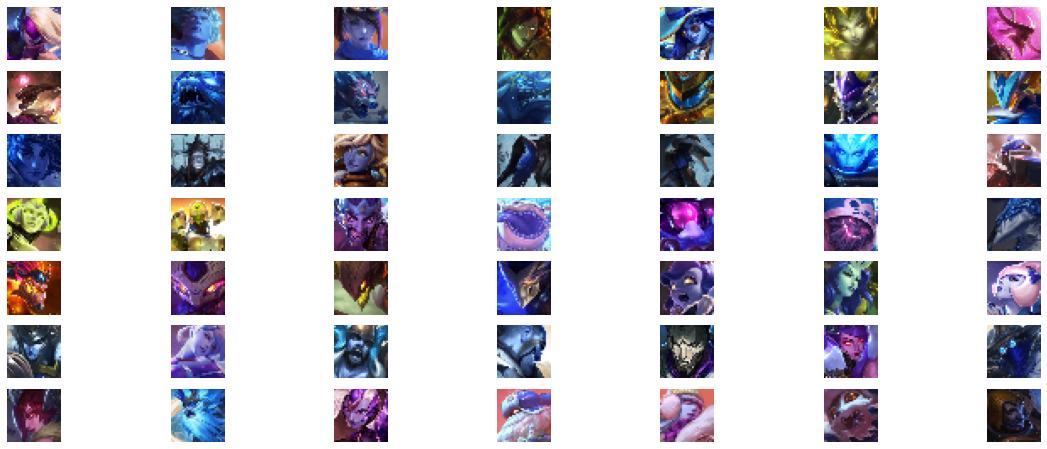# III. Image Data Generator¶

Define Image data generator

In [ ]:
datagen = ImageDataGenerator(
# Random rotation range and width and height and zoom in range
rotation_range=10,
width_shift_range=0.10,
height_shift_range=0.10,
shear_range=0.5,
zoom_range=0.10,
# Do not flip as 6,9 can be mis-recognized
horizontal_flip=False,
vertical_flip=False,
fill_mode='constant',
cval=0
)


Generating more trainning data and stack them to the old trainning array.

In [ ]:
# Array to store new image data
img_gen = []
# loop through all the training images, and for each of them, randomly generate more images
for img in trainX:
count = 0
# generating data until count reaches 15
for x_batch in datagen.flow(img.reshape((1,) + img.shape), batch_size=100):
img_gen.append(x_batch)
count += 1
if count >= 5:
break
# appending newly generated data to the training sets
trainX = np.vstack((trainX, img_gen))


Shape of the trainning data after generating more.

In [ ]:
trainX.shape

Out[ ]:
(8610, 32, 32, 3)

Display some of the generated image.

In [ ]:
for i in range(10):
cv2_imshow(cv2.resize(img_gen[i],(32,32)))
plt.show()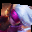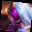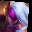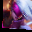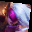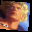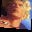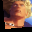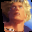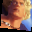# IV. Define and Train Discriminators¶

The first step is to define the discriminator model.

The model must take a sample image from our dataset as input and output a classification prediction as to whether the sample is real or fake. This is a binary classification problem.

• Inputs: Image with three color channel and width x height pixels in size.
• Outputs: Binary classification, likelihood the sample is real (or fake).

The discriminator model has a normal convolutional layer followed by three convolutional layers using a stride of 2×2 to downsample the input image. The model has no pooling layers and a single node in the output layer with the sigmoid activation function to predict whether the input sample is real or fake. The model is trained to minimize the binary cross entropy loss function, appropriate for binary classification.

We will use some best practices in defining the discriminator model, such as the use of LeakyReLU instead of ReLU, using Dropout, and using the Adam version of stochastic gradient descent with a learning rate of 0.0002 and a momentum of 0.5.

In [ ]:
# define the standalone discriminator model
def define_discriminator(in_shape=(32,32,3)):
model = Sequential()
# normal
# downsample
# downsample
# downsample
# classifier
# compile model
opt = Adam(lr=0.0002, beta_1=0.5)
model.compile(loss='binary_crossentropy', optimizer=opt, metrics=['accuracy'])
return model

# define model
model = define_discriminator()
# summarize the model
model.summary()
# plot the model
plot_model(model, to_file='discriminator_plot.png', show_shapes=True, show_layer_names=True)

Model: "sequential"
_________________________________________________________________
Layer (type)                 Output Shape              Param #
=================================================================
conv2d (Conv2D)              (None, 32, 32, 64)        1792
_________________________________________________________________
leaky_re_lu (LeakyReLU)      (None, 32, 32, 64)        0
_________________________________________________________________
conv2d_1 (Conv2D)            (None, 16, 16, 128)       73856
_________________________________________________________________
leaky_re_lu_1 (LeakyReLU)    (None, 16, 16, 128)       0
_________________________________________________________________
conv2d_2 (Conv2D)            (None, 8, 8, 128)         147584
_________________________________________________________________
leaky_re_lu_2 (LeakyReLU)    (None, 8, 8, 128)         0
_________________________________________________________________
conv2d_3 (Conv2D)            (None, 4, 4, 256)         295168
_________________________________________________________________
leaky_re_lu_3 (LeakyReLU)    (None, 4, 4, 256)         0
_________________________________________________________________
flatten (Flatten)            (None, 4096)              0
_________________________________________________________________
dropout (Dropout)            (None, 4096)              0
_________________________________________________________________
dense (Dense)                (None, 1)                 4097
=================================================================
Total params: 522,497
Trainable params: 522,497
Non-trainable params: 0
_________________________________________________________________

Out[ ]: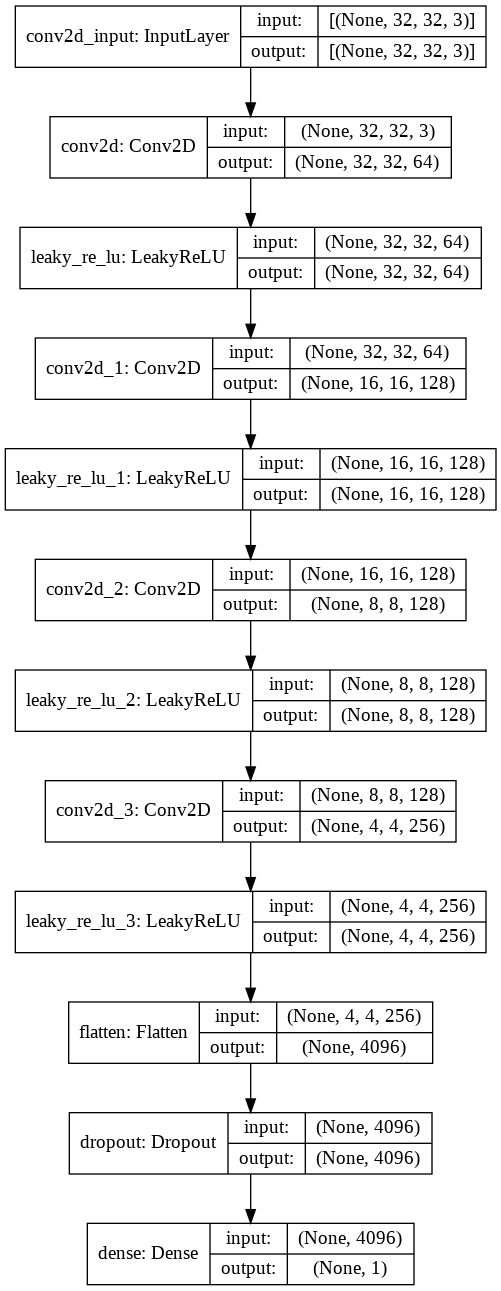We must scale the pixel values from the range of unsigned integers in [0,255] to the normalized range of [-1,1].

The generator model will generate images with pixel values in the range [-1,1] as it will use the tanh activation function, a best practice.

It is also a good practice for the real images to be scaled to the same range.

In [ ]:
# convert from unsigned ints to floats
X = trainX.astype('float32')
# scale from [0,255] to [-1,1]
X = (X - 127.5) / 127.5
# convert from unsigned ints to floats
X = trainX.astype('float32')
# scale from [0,255] to [-1,1]
X = (X - 127.5) / 127.5


The load_real_samples() function below implements the loading and scaling of real photographs.

In [ ]:
# load and prepare training images
# convert from unsigned ints to floats
X = trainX.astype('float32')
# scale from [0,255] to [-1,1]
X = (X - 127.5) / 127.5
return X


The model will be updated in batches, specifically with a collection of real samples and a collection of generated samples. On training, an epoch is defined as one pass through the entire training dataset.

In [ ]:
# select real samples
def generate_real_samples(dataset, n_samples):
# choose random instances
ix = randint(0, dataset.shape, n_samples)
# retrieve selected images
X = dataset[ix]
# generate 'real' class labels (1)
y = ones((n_samples, 1))
return X, y


We don’t have a generator model yet, so instead, we can generate images comprised of random pixel values, specifically random pixel values in the range [0,1], then scaled to the range [-1, 1] like our scaled real images.

The generate_fake_samples() function below implements this behavior and generates images of random pixel values and their associated class label of 0, for fake.

In [ ]:
# generate n fake samples with class labels
def generate_fake_samples(n_samples):
# generate uniform random numbers in [0,1]
X = rand(32 * 32 * 3 * n_samples)
# update to have the range [-1, 1]
X = -1 + X * 2
# reshape into a batch of color images
X = X.reshape((n_samples, 32, 32, 3))
# generate 'fake' class labels (0)
y = zeros((n_samples, 1))
return X, y


Finally, we need to train the discriminator model.

This involves repeatedly retrieving samples of real images and samples of generated images and updating the model for a fixed number of iterations.

We will ignore the idea of epochs for now.

In [ ]:
# train the discriminator model
def train_discriminator(model, dataset, n_iter=20, n_batch=32):
half_batch = int(n_batch / 2)
# manually enumerate epochs
for i in range(n_iter):
# get randomly selected 'real' samples
X_real, y_real = generate_real_samples(dataset, half_batch)
# update discriminator on real samples
_, real_acc = model.train_on_batch(X_real, y_real)
# generate 'fake' examples
X_fake, y_fake = generate_fake_samples(half_batch)
# update discriminator on fake samples
_, fake_acc = model.train_on_batch(X_fake, y_fake)
# summarize performance
print('>%d real=%.0f%% fake=%.0f%%' % (i+1, real_acc*100, fake_acc*100))


In this case, the discriminator model learns to tell the difference between real and randomly generated images very quickly, in about 20 batches.

In [ ]:
# define the discriminator model
model = define_discriminator()
# load image data
# fit the model
train_discriminator(model, dataset)

>1 real=25% fake=12%
>2 real=100% fake=0%
>3 real=100% fake=25%
>4 real=94% fake=75%
>5 real=100% fake=100%
>6 real=94% fake=100%
>7 real=100% fake=100%
>8 real=100% fake=100%
>9 real=94% fake=100%
>10 real=88% fake=100%
>11 real=100% fake=100%
>12 real=94% fake=100%
>13 real=94% fake=100%
>14 real=100% fake=100%
>15 real=88% fake=100%
>16 real=94% fake=100%
>17 real=100% fake=100%
>18 real=100% fake=100%
>19 real=100% fake=100%
>20 real=94% fake=100%


# V. Define Generator¶

The generator model is responsible for creating new, fake, but plausible small photographs of objects.

It does this by taking a point from the latent space as input and outputting a square color image.

The latent space is an arbitrarily defined vector space of Gaussian-distributed values, e.g. 100 dimensions. It has no meaning, but by drawing points from this space randomly and providing them to the generator model during training, the generator model will assign meaning to the latent points and, in turn, the latent space, until, at the end of training, the latent vector space represents a compressed representation of the output space, images, that only the generator knows how to turn into plausible images.

• Inputs: Point in latent space, e.g. a 100-element vector of Gaussian random numbers.
• Outputs: Two-dimensional square color image (3 channels) of 32 x 32 pixels with pixel values in [-1,1].
In [ ]:
def define_generator(latent_dim):
model = Sequential()
# foundation for 4x4 image
n_nodes = 256 * 4 * 4
# upsample to 8x8
# upsample to 16x16
# upsample to 32x32
# output layer
return model


We can see that, as designed, the first hidden layer has 4,096 parameters or 256 x 4 x 4, the activations of which are reshaped into 256 4 x 4 feature maps. The feature maps are then upscaled via the three Conv2DTranspose layers to the desired output shape of 32 x 32, until the output layer where three filter maps (channels) are created.

In [ ]:
# define the size of the latent space
latent_dim = 100
# define the generator model
model = define_generator(latent_dim)
# summarize the model
model.summary()
# plot the model
plot_model(model, to_file='generator_plot.png', show_shapes=True, show_layer_names=True)

Model: "sequential_2"
_________________________________________________________________
Layer (type)                 Output Shape              Param #
=================================================================
dense_2 (Dense)              (None, 4096)              413696
_________________________________________________________________
leaky_re_lu_8 (LeakyReLU)    (None, 4096)              0
_________________________________________________________________
reshape (Reshape)            (None, 4, 4, 256)         0
_________________________________________________________________
conv2d_transpose (Conv2DTran (None, 8, 8, 128)         524416
_________________________________________________________________
leaky_re_lu_9 (LeakyReLU)    (None, 8, 8, 128)         0
_________________________________________________________________
conv2d_transpose_1 (Conv2DTr (None, 16, 16, 128)       262272
_________________________________________________________________
leaky_re_lu_10 (LeakyReLU)   (None, 16, 16, 128)       0
_________________________________________________________________
conv2d_transpose_2 (Conv2DTr (None, 32, 32, 128)       262272
_________________________________________________________________
leaky_re_lu_11 (LeakyReLU)   (None, 32, 32, 128)       0
_________________________________________________________________
conv2d_8 (Conv2D)            (None, 32, 32, 3)         3459
=================================================================
Total params: 1,466,115
Trainable params: 1,466,115
Non-trainable params: 0
_________________________________________________________________

Out[ ]: